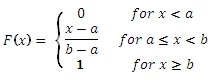# SQL Server uniform inverse distribution function

UNIFORMINV

Updated: 31 July 2010

Use UNIFORMINV to calculate the inverse of the lower cumulative distribution of the uniform distribution.

The formula for the lower cumulative distribution function is:Syntax
SELECT [devdb].[dbo].[UNIFORMINV] (
<@P, float,>
,<@Min, float,>
,<@Max, float,>)
Arguments
@P
is the value of the lower cumulative distribution function. @P is an expression of type float or of a type that implicitly converts to float
@Min
is the minimum value of the distribution. @Min is an expression of type float or of a type that implicitly converts to float
@Max
is the maximum value of the distribution. @Max is an expression of type float or of a type that implicitly converts to float
Return Types
float
Remarks
·         @P must be greater than or equal to zero and less than or equal to one (0 ≤ @P ≤ 1).
·         @Max must be greater than or equal to @Min (@Max > @Min).
Examples
Calculate the lower cumulative distribution function:
SELECT wct.UNIFORM(6,1,9,'True')
This produces the following result
----------------------
0.625

(1 row(s) affected)

Calculate the inverse of the lower cumulative distribution function:
SELECT wct.UNIFORMINV(0.625,1,9)

This produces the following result
----------------------
6

(1 row(s) affected)

### SupportCopyright 2008-2023 Westclintech LLC         Privacy Policy        Terms of Service